# How to extract data with specific text?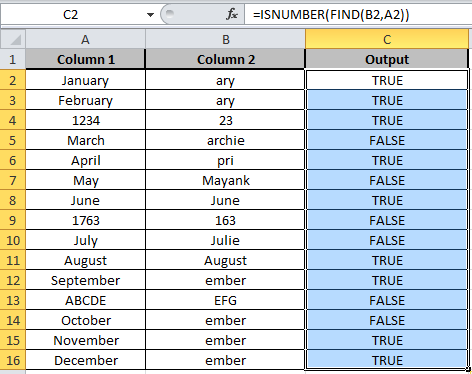In this article, we will learn How to extract data with specific text?

How to perform text operations in Excel ?

Text operation is used when we need to find some specific text data. For example, we need to find the separate first name, middle name and last name from the full name. This is done using the space separation on string values or text values. String operation are different from numerical values. So Excel also treats them differently.

String or Text formula in Excel

To search a string from a specific substring, we will use the ISNUMBER function along with the FIND function.

ISNUMBER function is used to check if the value in the cell contains a number or not.

Syntax of ISNUMBER:

 =ISNUMBER (value)

FIND function returns the location number of the character at which a specific character or text string is first found, reading left to right (case-sensitive).

Syntax of Find:

 =FIND(find_text,within_text,[start_num])

Example :

All of these might be confusing to understand. Let's understand how to use the function using an example.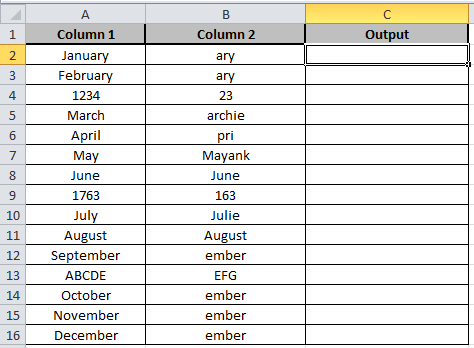Here we have two columns. Substring in Column B and Given string in Column A.

Write the formula in C2 cell

Formula:

 =ISNUMBER(FIND(B2,A2))

Explanation:

Find function takes the substring from the B2 cell of Column B and it then matches it with the given string in the A2 cell of Column A.

ISNUMBER checks if the string matches, it returns True else it returns False.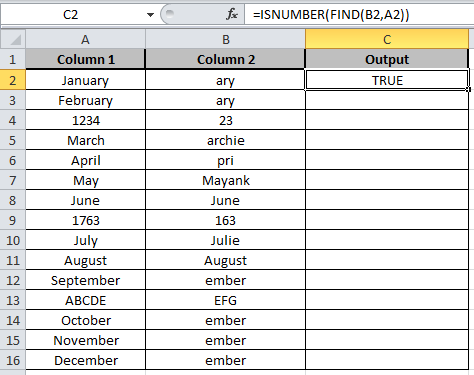Copy the formula in other cells, select the cells taking the first cell where the formula is already applied, use shortcut key Ctrl+ D.As you can see the output in column C shows True and False representing whether substring is there or not.

#### Alternate formula

There will be times when you would want to check if cell contains a specific text or not. We can do this by combining two functions, ISNUMBER and SEARCH or FIND function. Lets see some examples.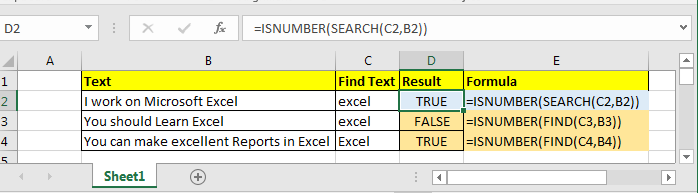#### Find Specific Text - Case Insensitive

We need an incentive function. A function that doesn’t care about upper or lowercase of alphabets. We will employ SEARCH function. He is cool about any case.

Generic Formula for Checking if cell contains Substring

 =ISNUMBER(SEARCH(“specific text”,”source text”)

In excel 2016, I have this list of strings.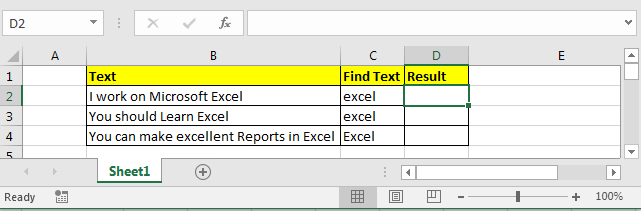Now I want to know if any cell contains substring “Excel” (case insensitive). If yes then print TRUE else FALSE.

I write this formula in Cell D2 and drag it down:

 =ISNUMBER(SEARCH(C2,B2))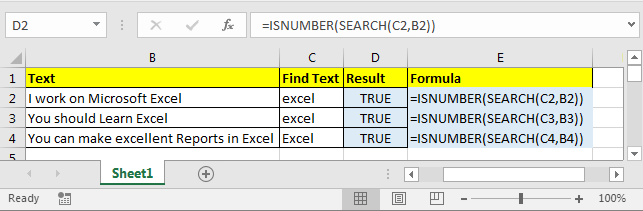We get all TRUE as each cell contains “Excel” or “excel” substring. Now lets see how to check for case sensitive cases.

#### Find Specific Text - Case Sensitive

We need a touchy function. The function that really sensitive about upper and lower cases and does not tolerate changes. FIND function is that touchy member of excel’s function family.

Generic Formula for Checking if cell contains substring

 =ISNUMBER(FIND(“specific text”,”source text”)

I want to know if any cell contains “Excel” not “excel”. It should print TRUE if and only if it finds “Excel”.

I write this formula in Cell D2 and drag it down:

 =ISNUMBER(FIND(C2,B2))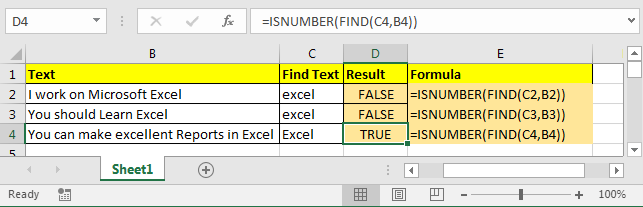In cell D2 and D3 you can see it return FALSE because B2 and B3 does not contains substring “excel”. IN cell D4 we are looking for “Excel” and which is found in String in Cell B4. Hence we have TRUE.

Explanation:

SEARCH and FIND both return location of found substring. If they found the given text then they return a number (first location of found text) else they return #VALUE! error.

ISNUMBER checks if supplied value is a number or not. If its a number it returns TRUE else FALSE.

If FIND or SEARCH find the specific text in cell, ISNUMBER returns TRUE else FALSE.

Using this result you can show customised out put using IF statement.

Excel formula if cell contains text then return value in another cell

 =IF(ISNUMBER(SEARCH(“specific text”,”source text”),print value if true, print value if false)

Hope this article about How to extract data with specific text is explanatory. Find more articles on calculating values and related Excel formulas here. If you liked our blogs, share it with your friends on Facebook. And also you can follow us on Twitter and Facebook. We would love to hear from you, do let us know how we can improve, complement or innovate our work and make it better for you. Write to us at info@exceltip.com.

Related Articles :

Excel REPLACE vs SUBSTITUTE function: The REPLACE and SUBSTITUTE functions are the most misunderstood functions. To find and replace a given text we use the SUBSTITUTE function. Where REPLACE is used to replace a number of characters in string…

Replace text from end of a string starting from variable position: To replace text from the end of the string, we use the REPLACE function. The REPLACE function uses the position of text in the string to replace.

How to Check if a string contains one of many texts in Excel: To find check if a string contains any of multiple text, we use this formula. We use the SUM function to add up all the matches and then perform a logic to check if the string contains any of the multiple strings.

Count Cells that contain specific text: A simple COUNTIF function will do the magic. To count the number of multiple cells that contain a given string we use the wildcard operator with the COUNTIF function.

Popular Articles :

How to use the IF Function in Excel : The IF statement in Excel checks the condition and returns a specific value if the condition is TRUE or returns another specific value if FALSE.

How to use the VLOOKUP Function in Excel : This is one of the most used and popular functions of excel that is used to lookup value from different ranges and sheets.

How to Use SUMIF Function in Excel : This is another dashboard essential function. This helps you sum up values on specific conditions.

How to use the COUNTIF Function in Excel : Count values with conditions using this amazing function. You don't need to filter your data to count specific values. Countif function is essential to prepare your dashboard.

Terms and Conditions of use

The applications/code on this site are distributed as is and without warranties or liability. In no event shall the owner of the copyrights, or the authors of the applications/code be liable for any loss of profit, any problems or any damage resulting from the use or evaluation of the applications/code.